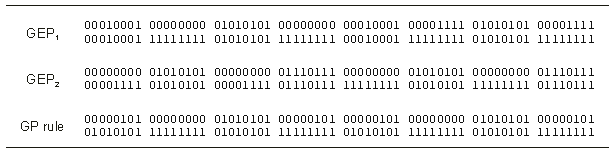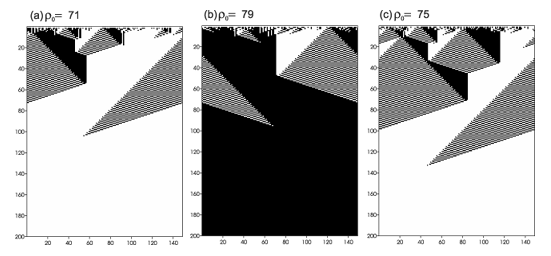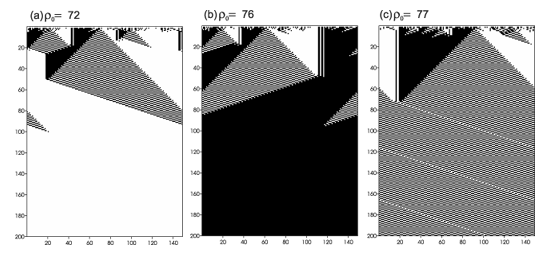GEP Book

Home
News
Author
Q&A
Tutorials
GEP Biblio
Contacts

Visit Gepsoft

 C. FERREIRA Complex Systems, 13 (2): 87-129, 2001

Gene Expression Programming: A New Adaptive Algorithm for Solving Problems

Two Gene Expression Programming Discovered Rules

In one experiment F = {A, O, N, I} (“A” represents the boolean function AND, “O” represents OR, “N” represents NOT, and “I” stands for IF) and T = {c, b, a, u, 1, 2, 3}. The parameters used per run are shown in Table 4, column 1.

Table 4

 GEP1 GEP2 Number of generations 50 50 Population size 30 50 Number of ICs 25 100 Head length 17 4 Number of genes 1 3 Chromosome length 52 39 Mutation rate 0.038 0.051 One-point recombination rate 0.5 0.7 IS transposition rate 0.2 -- IS elements length 1,2,3 -- RIS transposition rate 0.1 -- RIS elements length 1,2,3 --

The fitness was evaluated against a set of 25 unbiased ICs (i.e., ICs with equal probability of having a 1 or a 0 at each cell). In this case, the fitness is a function of the number of ICs i for which the system stabilizes correctly to a configuration of all 0s or 1s after 2xN time steps, and it was designed in order to privilege individuals capable of correctly classifying ICs both with a majority of 1s and 0s. Thus, if the system converged, in all cases, indiscriminately to a configuration of 1s or 0s, only one fitness point was attributed. If, in some cases, the system correctly converged either to a configuration of 0s or 1s, f = 2. In addition, rules converging to an alternated pattern of all 1s and all 0s were eliminated, as they are easily discovered and invade the populations impeding the discovery of good rules. And finally, when an individual program could correctly classify ICs both with majorities of 1s and 0s, a bonus equal to the number of ICs C was added to the number of correctly classified ICs, being in this case f = i + C. For instance, if a program correctly classified two ICs, one with a majority of 1s and another with a majority of 0s, it receives 2 + 25 = 27 fitness points.

In this experiment a total of 7 runs were made. In generation 27 of run 5, an individual evolved with fitness 44:

0123456789012345678901234567890123456789012345678901
OAIIAucONObAbIANIb1u23u3a12aacb3bc21aa2baabc3bccuc13

Note that the ORF ends at position 28. This program has an accuracy of 0.82513 tested over 100,000 unbiased ICs in a 149x298 lattice, thus better than the 0.824 of the GP rule tested in a 149x320 lattice [16, 17]. The rule table of this rule (GEP1) is shown in Table 5. Figure 15 shows three space-time diagrams for this new rule.

Table 5

Description of the two new rules (GEP1 and GEP2) discovered using GEP for the density-classification problem. The GP rule is also shown. The output bits are given in lexicographic order starting with 0000000 and finishing with 1111111.As a comparison, GP used populations of 51,200 individuals and 1000 ICs for 51 generations , thus a total of 51,200 x 1,000 x 51 = 2,611,200,000 fitness evaluations were made, whereas GEP only made 30 x 25 x 50 = 37,500 fitness evaluations. Therefore, in this problem, GEP outperforms GP by more than four orders of magnitude (69,632 times).Figure 15. Three space-time diagrams describing the evolution of CA states for the GEP1 rule. The number of 1s in the IC (r0) is shown above each diagram. In (a) and (b) the CA correctly converged to a uniform pattern; in (c) it converged wrongly to a uniform pattern.

In another experiment, a rule slightly better than GEP1, with an accuracy of 0.8255, was obtained. Again, its performance was determined over 100,000 unbiased ICs in a 149x298 lattice. In this case F = {I, M} (“I” stands for IF, and “M” represents the majority function with three arguments), and T was obviously the same. In this case, a total of 100 unbiased ICs and three-genic chromosomes with sub-ETs linked by IF were used. The parameters used per run are shown in the second column of Table 4.

The fitness function was slightly modified by introducing a ranking system, where individuals capable of correctly classifying between 2 and 3/4 of the ICs receive one bonus equal to C; if between 3/4 and 17/20 of the ICs are correctly classified two bonus C; and if more than 17/20 of the ICs are correctly classified three bonus C. Also, in this experiment, individuals capable of correctly classifying only one kind of situation, although not indiscriminately, were differentiated and had a fitness equal to i.

By generation 43 of run 10, an individual evolved with fitness 393:

012345678901201234567890120123456789012
MIuua1113b21cMIM3au3b2233bM1MIacc1cb1aa

Its rule table is shown in Table 5. Figure 16 shows three space-time diagrams for this new rule (GEP2). Again, in this case the comparison with GP shows that GEP outperforms GP by a factor of 10,444.Figure 16. Three space-time diagrams describing the evolution of CA states for the GEP2 rule. The number of 1s in the IC (r0) is shown above each diagram. In (a) and (b) the CA converges, respectively, to the correct configuration of all 0s and all 1s; in (c) the CA could not converge to a uniform pattern.

Home | Contents | Previous | Next